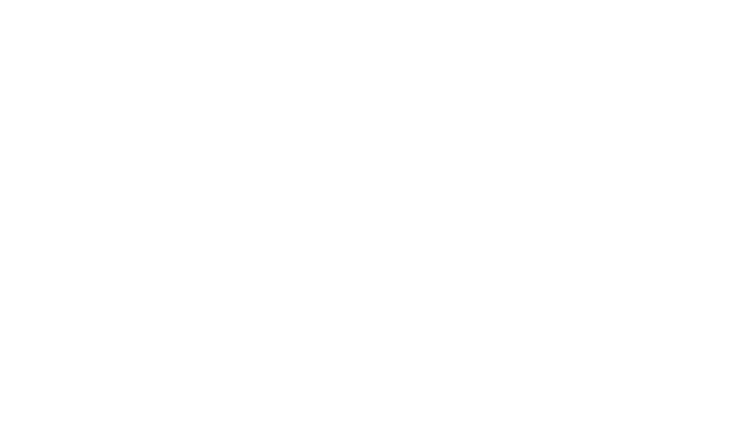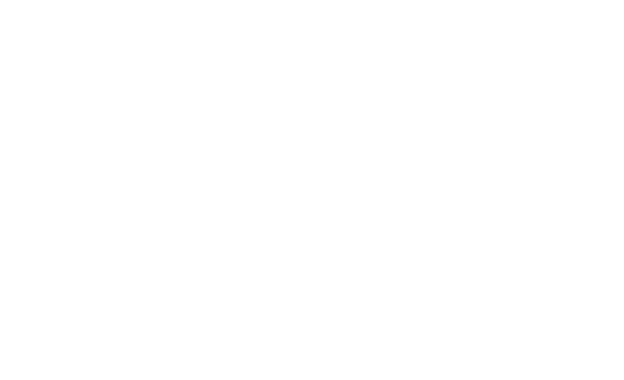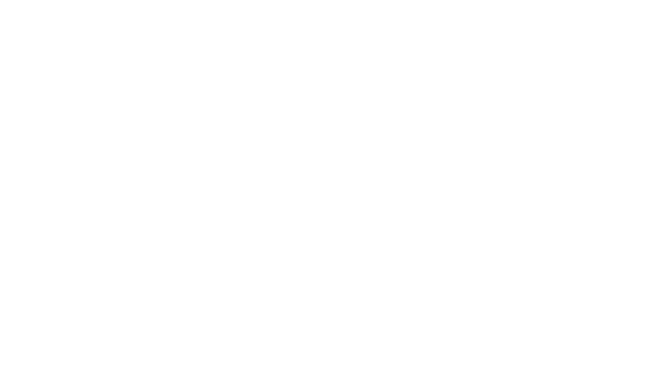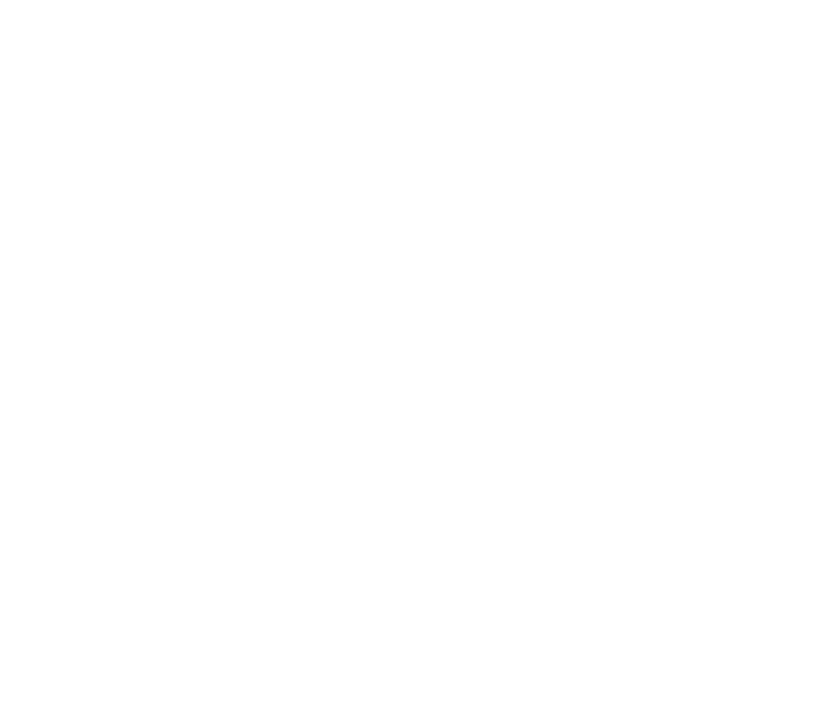# Virtual base class in C++ OOP

## What is a Virtual base class?

The virtual base class is very helpful in virtual inheritance.

When we use multiple inheritances, then multiple “instances” of a given class appearing in an inheritance hierarchy. It leads to redundancy. Redundancy is not good in such a scenario.

When we declare a class as a virtual class, then it means that we are helping the compiler that we only required a single instance.

## Why we use a virtual base class?

Virtual base class means that child classes can access the base classes virtually from a long distance in the parent-child hierarchy.

## How to declare a class as a virtual base class?

class A {
public:
int number;
A()
{
int number = 10;
}
};
class B : public virtual A {};
class C : public virtual A {};
class D : public B, public C{};

Explanation of Diagrams

Class A:Base Parent Class

Class B:  B is child class of class A.

Class C: C is a child class of class A.

Class D: D is a child class of class B and Class C. A is the grandfather of class D.

Step 1:

1. Create class A
2. declare the variable “int number” inside class A.
3. Class C and Class B get the “int number” variable from class A.
4. Class D is getting the “int number” from both class B, and Class C. This is not good to do.We must avoid this redundancy and never inherit the same member(e.g int number) from multiple parent classes.The solution to solve the above-mentioned problem is to make the parent base class a virtual class.Here, Class D is getting the “int number” directly from the parent base virtual class.

## C++ Program  of Virtual base class

Let us code the above-mentioned scenario with C++.

```#include <iostream>
using namespace std;
class A {
public:
int number;
A() // constructor
{
number = 10;
}
};
class B : public virtual A {};
class C : public virtual A {};
class D : public B, public C{};
int main()
{
D object; // object is created for class d
cout << "number = " << object.number << endl;
return 0;
}```

Output

number=3## Example 2 with error

```#include <iostream>
using namespace std;
class T4TUTORIALS_ONE {
public: int n1;
};

class T4TUTORIALS_TWO : public T4TUTORIALS_ONE {
public: int n2;
};

class T4TUTORIALS_THREE : public T4TUTORIALS_ONE {
public: int n3;
};

class T4TUTORIALS_FOUR : public T4TUTORIALS_TWO, public T4TUTORIALS_THREE {
public: int n4;
};
int main() {
T4TUTORIALS_FOUR obj;
obj.n1 = 40; //error
obj.n1 = 30; // error
obj.n2 = 60;
obj.n3 = 70;
obj.n4 = 80;
cout<< " T4TUTORIALS_ONE : "<< obj.n1	// error
cout<< " T4TUTORIALS_TWO : "<< obj.n2;
cout<< " T4TUTORIALS_THREE: "<< obj.n3;
cout<< " T4TUTORIALS_FOUR: "<< obj.n4;
}

```

## Example 2 without error

```#include <iostream>
using namespace std;
class T4TUTORIALS_ONE {
public: int n1;
};

class T4TUTORIALS_TWO : virtual public T4TUTORIALS_ONE {
public: int n2;
};

class T4TUTORIALS_THREE : virtual public T4TUTORIALS_ONE {
public: int n3;
};

class T4TUTORIALS_FOUR : public T4TUTORIALS_TWO, public T4TUTORIALS_THREE {
public: int n4;
};
int main() {
T4TUTORIALS_FOUR obj;
obj.n1 = 40;
obj.n1 = 30;
obj.n2 = 60;
obj.n3 = 70;
obj.n4 = 80;
cout<< " T4TUTORIALS_ONE : "<< obj.n1;
cout<< " T4TUTORIALS_TWO : "<< obj.n2;
cout<< " T4TUTORIALS_THREE: "<< obj.n3;
cout<< " T4TUTORIALS_FOUR: "<< obj.n4;
}```

## Virtual Base Class Exercise Solution

1. Write a C++ Program to display the reverse of a number using the Virtual base Class in Object Oriented Programming(OOP).
2. Write a program in C++ to convert a decimal number into binary without using an array and using the Virtual base Class in Object Oriented Programming(OOP).
3. Write a C++ program to find HCF (Highest Common Factor) of two numbers using the Virtual base Class in Object Oriented Programming(OOP).
4. C++ program to print rhombus or parallelogram star pattern using the Virtual base Class in Object Oriented Programming(OOP).
5. Write a C program to check whether a number is a Strong Number or not using the Virtual base Class in Object Oriented Programming(OOP).
6. Write a program in C++ to find the prime numbers within a range of numbers by using the Virtual base Class in Object Oriented Programming(OOP).
7. Write a program in C++ to convert a decimal number into octal without using an array and Virtual base Class in Object Oriented Programming(OOP).
8. Write a C++ program to display Pascal’s triangle using Virtual base Class in Object Oriented Programming(OOP).
9. C++ program to print hollow rhombus, parallelogram star pattern using the Virtual Base Class.
10. C++ program to print mirrored right triangle star pattern using virtual base class.
11. Write a program in C++ to display the sum of the series by using the virtual base class.
12. Write a C++ program to find Strong Numbers within a range of numbers by using the virtual base class.
13. Write a program in C++ to display the cube of the number up to a given integer by using the virtual base class.
14. Write a program in C++ to check Armstrong number of n digits by using the virtual base class.
15. Write a program in C++ to print a string in reverse order by using the virtual base class.
16. Write a C++ program to find the perfect numbers within a given number of range by using the virtual base class.
17. Write a C++ Program to display the pattern like a pyramid using the alphabet by using the virtual base class. Solution
18. Write a program in C++ to display the n terms of odd natural number and their sum. Solution
19. Write a program in C++ to make such a pattern like a pyramid with a number that will repeat the number in the same row.
20. Write a program in C++  to Check Whether a Number can be Express as Sum of Two Prime Numbers by using the virtual base class.
21. Develop a C++ program to print a hollow square or rectangle star pattern by using the virtual base class.
22. Write a program in C++ to convert an Octal number to a Decimal number by using the virtual base class.
23. Write a program in C++ to find the number and sum of all integer between 100 and 200 which are divisible by 9.
24. Write a program in C++ to make such a pattern like a pyramid with numbers increased by 1 by using the virtual base class.
25. C++ program to print the hollow right triangle star pattern by using the virtual base class in object-oriented programming.
26. Write a C++ program to find out the sum of an A.P. series by using the virtual base class.
27. Write a program in C++ to display the pattern like a diamond by using the virtual base class.
28. Fibonacci Series Program in C++ with virtual inheritance.